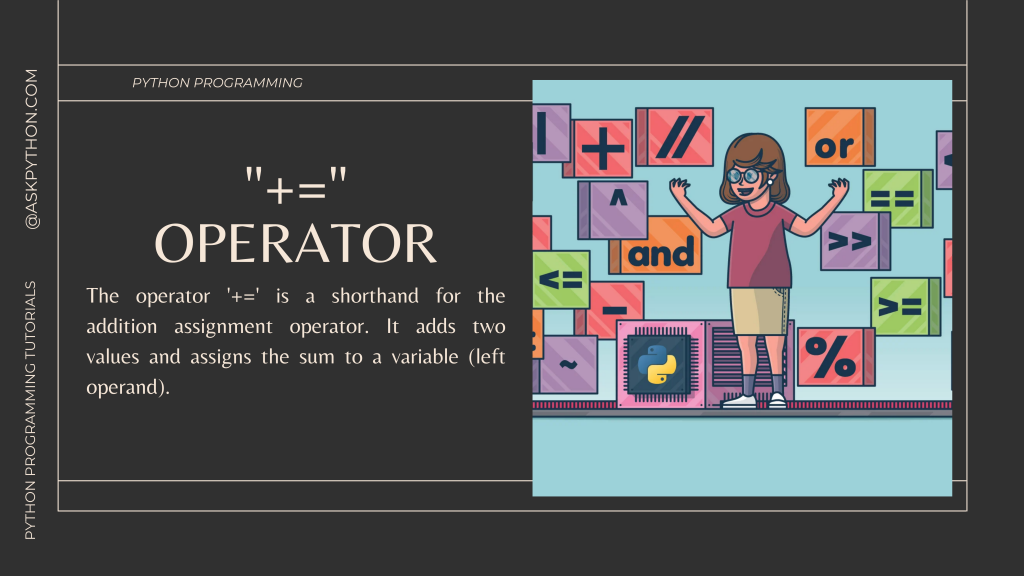# The += Operator In Python – A Complete GuideIn this lesson, we will look at the += operator in Python and see how it works with several simple examples.

The operator ‘+=’ is a shorthand for the addition assignment operator. It adds two values and assigns the sum to a variable (left operand).

Let’s look at three instances to have a better idea of how this operator works.

## 1. Adding Two Numeric Values With += Operator

In the code mentioned below, we have initialized a variable X with an initial value of 5 and then add value 15 to it and store the resultant value in the same variable X.

```X = 5
print("Value Before Change: ", X)
X += 15
print("Value After Change: ", X)
```

The output of the Code is as follows:

```Value Before Change:  5
Value After Change:  20
```

```S1 = "Welcome to "

print("First String : ", S1)
print("Second String: ", S2)
S1+=S2
print("Final String: ", S1)
```

In the code mentioned above, we initialized two variables S1 and S2 with initial values as “Welcome to ” and ”AskPython” respectively.

We then add the two strings using the ‘+=’ operator which will concatenate the values of the string.

The output of the code is as follows:

```First String :  Welcome to
```

## 3. Understanding Associativity of “+=” operator in Python

The associativity property of the ‘+=’ operator is from right to left. Let’s look at the example code mentioned below.

```X = 5
Y = 10
X += Y>>1
print(X)
```

We initialized two variables X and Y with initial values as 5 and 10 respectively. In the code, we right shift the value of Y by 1 bit and then add the result to variable X and store the final result to X.

The output comes out to be X = 10 and Y = 10.

## Conclusion

Congratulations! You just learned about the ‘+=’ operator in python and also learned about its various implementations.

Liked the tutorial? In any case, I would recommend you to have a look at the tutorials mentioned below:

Thank you for taking your time out! Hope you learned something new!! 😄